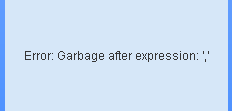# QlikView App Development

Discussion Board for collaboration related to QlikView App Development.

Highlighted
Contributor III

## Use of NUM in set analysis combined with aggr

Hello

I have 2 expressions that I would like to add together and then use the NUM function to format.

The below works fine and give me a value of -123.

=Sum(Aggr(
If(Sum({<proceeds = {'Proceeds'},period = {">=\$(vStartPeriod_CY)<=\$(vEndPeriod_CY)"}>} [GPR GBP]) * -1 > 500000, Round(Sum({<proceeds = {'Proceeds'},period = {">=\$(vStartPeriod_CY)<=\$(vEndPeriod_CY)"}>} [GPR GBP]) * -1, 1E6))
,
bussline_reclass, portfolio, portfolio_text))/1000000
+
floor(sum({
<
moves = {"ICADDS", "ICSYND"}, gross_investment = {"Gross investment"}, period = {">=\$(vStartPeriod_CY)<=\$(vEndPeriod_CY)"}
> }
[Investment GBP]) +500000,1000000 )/1000000 *-1

I would like to show the value as (123) so I used the NUM function

I therefore did the below

=NUM(Sum(Aggr(
If(Sum({<proceeds = {'Proceeds'},period = {">=\$(vStartPeriod_CY)<=\$(vEndPeriod_CY)"}>} [GPR GBP]) * -1 > 500000, Round(Sum({<proceeds = {'Proceeds'},period = {">=\$(vStartPeriod_CY)<=\$(vEndPeriod_CY)"}>} [GPR GBP]) * -1, 1E6))
,
bussline_reclass, portfolio, portfolio_text))/1000000
+
floor(sum({
<
moves = {"ICADDS", "ICSYND"}, gross_investment = {"Gross investment"}, period = {">=\$(vStartPeriod_CY)<=\$(vEndPeriod_CY)"}
> }
[Investment GBP]) +500000,1000000 )/1000000 *-1
)
,'#,##0;(#,##0)'

I get expression ok in the expression box but then the error below.  Can anyone explain what I am doing wrong?Many thanks

Paul

Tags (3)
5 Replies
Honored Contributor II

## Re: Use of NUM in set analysis combined with aggr

try this:

```=NUM((Sum(Aggr(If(Sum({<proceeds = {'Proceeds'},period = {">=\$(vStartPeriod_CY)<=\$(vEndPeriod_CY)"}>} [GPR GBP]) * -1 > 500000,Round(Sum({<proceeds = {'Proceeds'},period = {">=\$(vStartPeriod_CY)<=\$(vEndPeriod_CY)"}>} [GPR GBP]) * -1, 1E6)), bussline_reclass, portfolio, portfolio_text))/1000000+floor(sum({<moves = {"ICADDS", "ICSYND"}, gross_investment = {"Gross investment"}, period = {">=\$(vStartPeriod_CY)<=\$(vEndPeriod_CY)"}> }[Investment GBP]) +500000,1000000 )/1000000 *-1 ),'#,##0')

```
Contributor III

## Re: Use of NUM in set analysis combined with aggr

Hi Frank

That gave me -123.  What I want is if the number is positive for it to be 123 but if negative (123) thanksMVP

## Re: Use of NUM in set analysis combined with aggr

May be this

=Num(Sum(Aggr(

If(Sum({<proceeds = {'Proceeds'},period = {">=\$(vStartPeriod_CY)<=\$(vEndPeriod_CY)"}>} [GPR GBP]) * -1 > 500000,Round(Sum({<proceeds = {'Proceeds'},period = {">=\$(vStartPeriod_CY)<=\$(vEndPeriod_CY)"}>} [GPR GBP]) * -1, 1E6))

, bussline_reclass, portfolio, portfolio_text))/1000000

+

floor(sum({

<moves = {"ICADDS", "ICSYND"}, gross_investment = {"Gross investment"}, period = {">=\$(vStartPeriod_CY)<=\$(vEndPeriod_CY)"}

> }[Investment GBP]) +500000,1000000 )/1000000 *-1

,'#,##0;(#,##0)')

Contributor III

## Re: Use of NUM in set analysis combined with aggr

Actually Frank, using your logic I have worked it out.  It is

=NUM((Sum(Aggr
If(Sum({<proceeds = {'Proceeds'},period = {">=\$(vStartPeriod_CY)<=\$(vEndPeriod_CY)"}>} [GPR GBP]) * -1 > 500000,Round(Sum({<proceeds = {'Proceeds'},period = {">=\$(vStartPeriod_CY)<=\$(vEndPeriod_CY)"}>} [GPR GBP]) * -1, 1E6))
,
bussline_reclass, portfolio, portfolio_text))/1000000

floor(sum({
<
moves = {"ICADDS", "ICSYND"}, gross_investment = {"Gross investment"}, period = {">=\$(vStartPeriod_CY)<=\$(vEndPeriod_CY)"
> }
[Investment GBP]) +500000,1000000 )/1000000 *-1

,'#,##0;(#,##0)')

Valued Contributor

## Re: Use of NUM in set analysis combined with aggr

1. =NUM((Aggr(Sum(
2. If(Sum({<proceeds = {'Proceeds'},period = {">=\$(vStartPeriod_CY)<=\$(vEndPeriod_CY)"}>} [GPR GBP]) * -1 > 500000,Round(Sum({<proceeds = {'Proceeds'},period = {">=\$(vStartPeriod_CY)<=\$(vEndPeriod_CY)"}>} [GPR GBP]) * -1, 1E6))
3. , bussline_reclass, portfolio, portfolio_text))/1000000
4. floor(sum({
5. <moves = {"ICADDS", "ICSYND"}, gross_investment = {"Gross investment"}, period = {">=\$(vStartPeriod_CY)<=\$(vEndPeriod_CY)"}
6. > }[Investment GBP]) +500000,1000000 )/1000000 *-1
7. ,'#,##0')